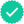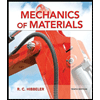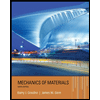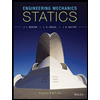#### Concept explainers#### Videos

QuestionChapter 6.1, Problem 1P
To determine

## The local component of acceleration in x direction.The convective component of acceleration in x direction.The local component of acceleration in y direction.The convective component of acceleration in y direction.The magnitude and direction of velocity at x=y=2 ft and time t=0.The magnitude of acceleration at x=y=2 ft and time t=0.The direction of acceleration at x=y=2 ft and time t=0.The local component of acceleration in x direction is 2x.

The local component of acceleration in y direction is 2y.

The convective component of acceleration in x direction is 4xt2.

The convective component of acceleration in y direction is 4yt2.

The magnitude of acceleration at x=y=2ft and time t=0 is 5.66ft/s.

The direction of acceleration at x=y=2ft and time t=0 is 45°.

### Explanation of Solution

Write the expression of velocity in a certain two dimensional flow field.

V=2xti^2ytj^                                                                                           (I)

Here, the displacement in x direction is x, the displacement in y direction is y, the time is t, the unit vector in x direction is i^ and the unit vector in y direction is j^.

Write the expression of velocity.

V=ui^+vj^                                                                                                (II)

Here, the x component of velocity is u and the y component of the velocity is v.

Compare the term of unit vector from Equation (I) and (II).

u=2xt                                                                                                      (III)

v=2yt                                                                                                    (IV)

Write the expression of local component of acceleration in x direction.

(ax)Local=ut                                                                                         (V)

Write the expression of local component of acceleration in y direction.

(ay)Local=vt                                                                                         (VI)

Write the expression of convective acceleration in x direction.

(ax)Convective=uux+vuy                                                                     (VII)

Write the expression of convective acceleration in y direction.

(ay)Convective=uvx+vvy                                                                     (VIII)

Write the expression of acceleration.

a={(ax)Local+(ax)Convection}i^+{(ay)Local+(ay)Convection}j^                       (IX)

Substitute 2x for (ax)Local, 2y for (ay)Local, 4xt2 for (ax)Convection and 4yt2 for (ay)Convection in Equation (IX).

a={2x+4xt2}i^+{2y+4yt2}j^                                                      (X)

Write the expression of acceleration.

a=axi^+ayj^                                                                                      (XI)

Here, the acceleration component in x direction is ax and the acceleration component in y direction is ay.

Write the expression of direction of acceleration.

θ=tan1(ayax)                                                                            (XII)

Write the expression of magnitude of the acceleration.

a=ax2+ay2                                                                             (XIII)

Conclusion:

Substitute 2xt for u in Equation (V).

(ax)Local=t(2xt)=2x×1=2x

Thus, the local component of acceleration in x direction is 2x.

Substitute 2yt for v in Equation (VI).

(ay)Local=t(2yt)=2y×1=2y

Thus, the local component of acceleration in y direction is 2y.

Substitute 2xt for u in Equation (VII).

(ax)Convective=(2xt)x(2xt)+(2yt)y(2xt)=(2xt)×2t×1(2yt)×2t×0=4xt2

Thus, the convective component of acceleration in x direction is 4xt2.

Substitute 2yt for v in Equation (VIII).

(ax)Convective=(2xt)x(2yt)+(2yt)y(2yt)=(2xt)×2t×0(2yt)×(2t)×1=4yt2

Thus, the convective component of acceleration in y direction is 4yt2.

Substitute 2ft for x and 0 for t in Equation (III).

u=2×(2ft)×0=0

Substitute 2ft for y and 0 for t in Equation (IV).

y=2×(2ft)×0=0

Substitute 0 for u and 0 for v in Equation (V).

V=0i^+0j^=0

Thus, The magnitude and direction of velocity at x=y=2ft and time t=0 is 0.

Substitute 2ft for x, 2ft for y and 0 for t in Equation (X).

a={2(2ft)+4(2ft)×0}i^+{2(2ft)+4(2ft)×0}j^=(4ft)i^(4ft)j^                            (XIV)

Compare the unit vector of Equation (XI) and (XIV).

ax=4ft/s

ay=4ft/s

Substitute 4ft/s for ax and 4ft/s for ay in Equation (XIII).

a=(4ft/s)2+(4ft/s)2=(16ft2/s2)+(16ft2/s2)=(32ft2/s2)=5.66ft/s

Thus, the magnitude of acceleration at x=y=2ft and time t=0 is 5.66ft/s.

Substitute 4ft/s for ax and 4ft/s for ay in Equation (XII).

θ=tan1(4ft/s4ft/s)=tan1(1)=45°

Thus, the direction of acceleration at x=y=2ft and time t=0 is 45°.

### Want to see more full solutions like this?

Subscribe now to access step-by-step solutions to millions of textbook problems written by subject matter experts!
10:54
Knowledge BoosterRecommended textbooks for you
•Elements Of Electromagnetics
Mechanical Engineering
ISBN:9780190698614
Publisher:Oxford University PressMechanics of Materials (10th Edition)
Mechanical Engineering
ISBN:9780134319650
Author:Russell C. Hibbeler
Publisher:PEARSONThermodynamics: An Engineering Approach
Mechanical Engineering
ISBN:9781259822674
Author:Yunus A. Cengel Dr., Michael A. Boles
Publisher:McGraw-Hill Education
•Control Systems Engineering
Mechanical Engineering
ISBN:9781118170519
Author:Norman S. Nise
Publisher:WILEYMechanics of Materials (MindTap Course List)
Mechanical Engineering
ISBN:9781337093347
Author:Barry J. Goodno, James M. Gere
Publisher:Cengage LearningEngineering Mechanics: Statics
Mechanical Engineering
ISBN:9781118807330
Author:James L. Meriam, L. G. Kraige, J. N. Bolton
Publisher:WILEY
•Elements Of Electromagnetics
Mechanical Engineering
ISBN:9780190698614
Publisher:Oxford University PressMechanics of Materials (10th Edition)
Mechanical Engineering
ISBN:9780134319650
Author:Russell C. Hibbeler
Publisher:PEARSONThermodynamics: An Engineering Approach
Mechanical Engineering
ISBN:9781259822674
Author:Yunus A. Cengel Dr., Michael A. Boles
Publisher:McGraw-Hill EducationControl Systems Engineering
Mechanical Engineering
ISBN:9781118170519
Author:Norman S. Nise
Publisher:WILEYMechanics of Materials (MindTap Course List)
Mechanical Engineering
ISBN:9781337093347
Author:Barry J. Goodno, James M. Gere
Publisher:Cengage LearningEngineering Mechanics: Statics
Mechanical Engineering
ISBN:9781118807330
Author:James L. Meriam, L. G. Kraige, J. N. Bolton
Publisher:WILEY